# Divide by 3 Printable Worksheets

Hey there viewers! In this edition, we bring to you this division worksheet to get your kids started with learning division. Since the word division is derived from “divide”, which means to separate things into halves, division can be defined as the process of splitting large numbers into equal smaller numbers. It is one of the four foundational exercises in mathematics and is denoted by the “/” sign.

It is an arithmetic operation in which numbers are combined and divided to form a new number. Let us take a look at some of the common terms used in division.

• Dividend- The number that is divided is called the dividend.
• Divisor-The number by which we divide is called the divisor.
• Quotient-The result that we get after division is the quotient
• Remainder- The number left after division is the remainder.

Thus, when we perform division, we divide a dividend with a divisor and the quotient is the desired result. There will be no remainder if the dividend is perfectly divisible by the divisor.

The worksheet presents kids with several different questions. Children would practice their division skills as they’ll divide the numbers by three (3). Children should be familiar with the rule of divisibility for the number 3 to solve this worksheet easily.

## The rule of divisibility for 3-

According to the divisibility rule for the number 3, if the sum of the dividend is divisible by 3, the number is perfectly divisible by 3. Ex: The sum of the two digits of the number 69 is 15 which is divisible by 3, hence 69 is divisible by 3, on the other hand, the sum of the two digits of 67 is 13 which is not perfectly divisible by 3 hence it 67 is not divisible by 3.

Given in the following worksheet are several questions that students need to attempt.  Make sure they write their name, date, teacher’s name and section before attempting the questions.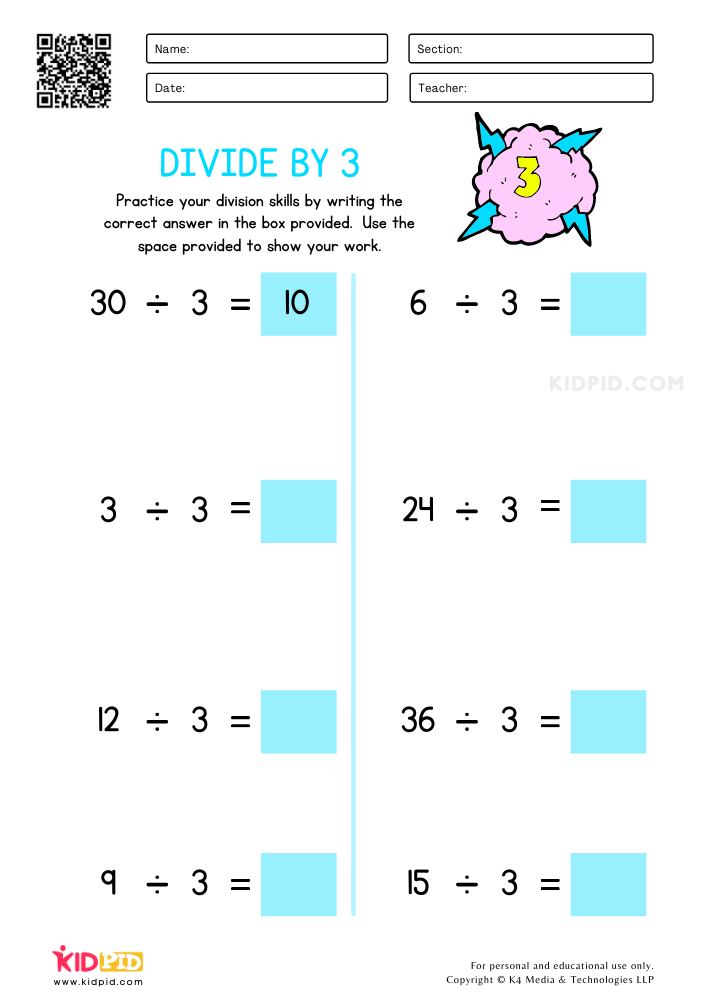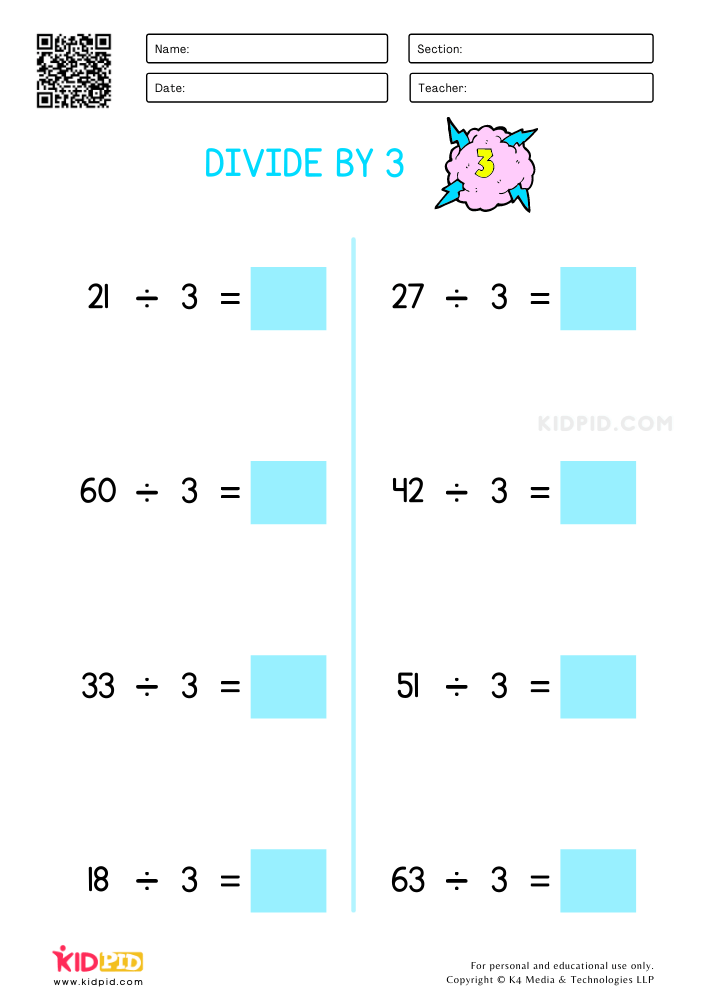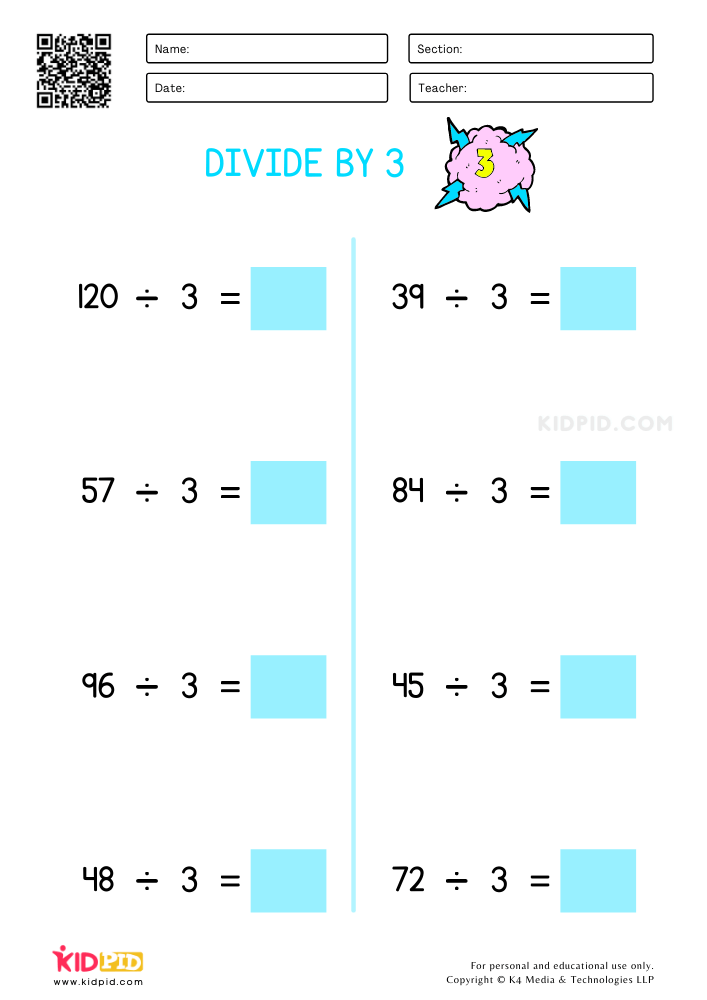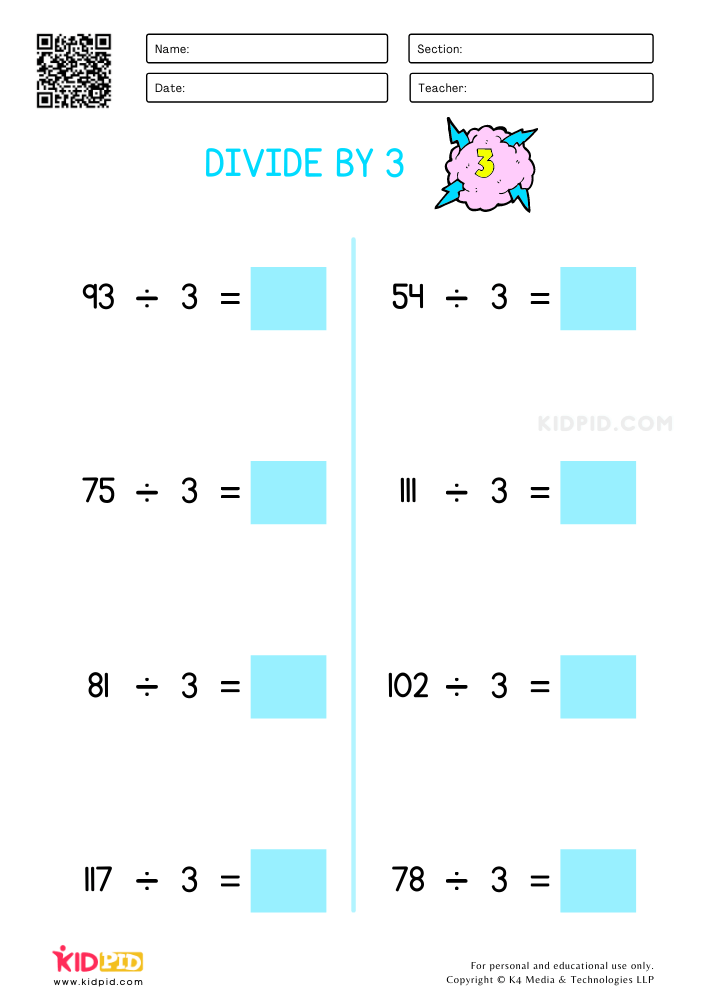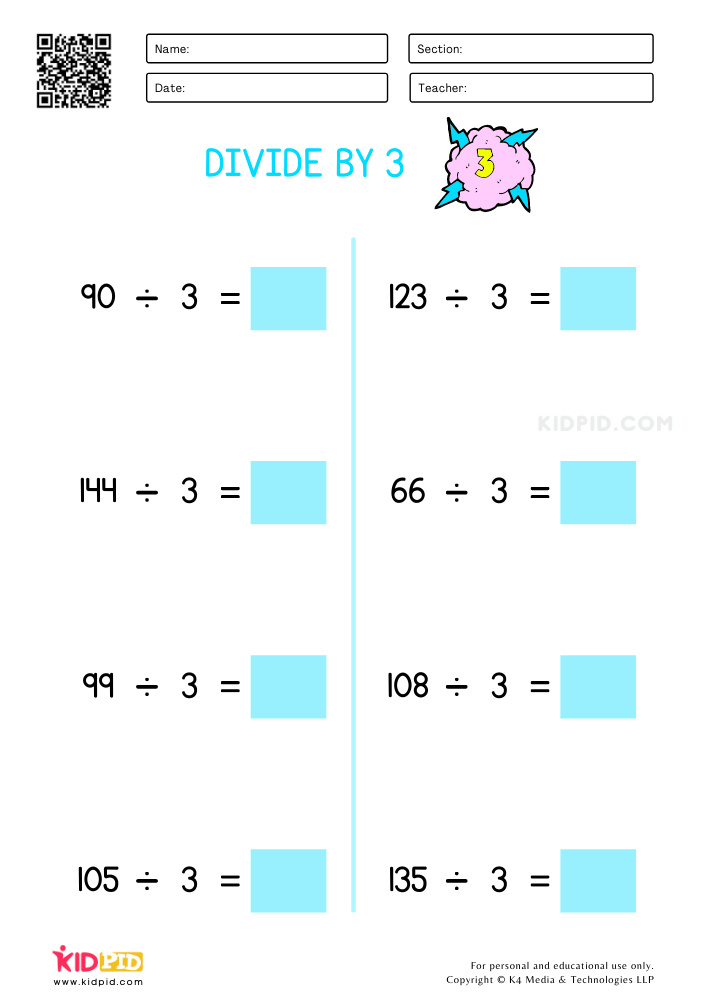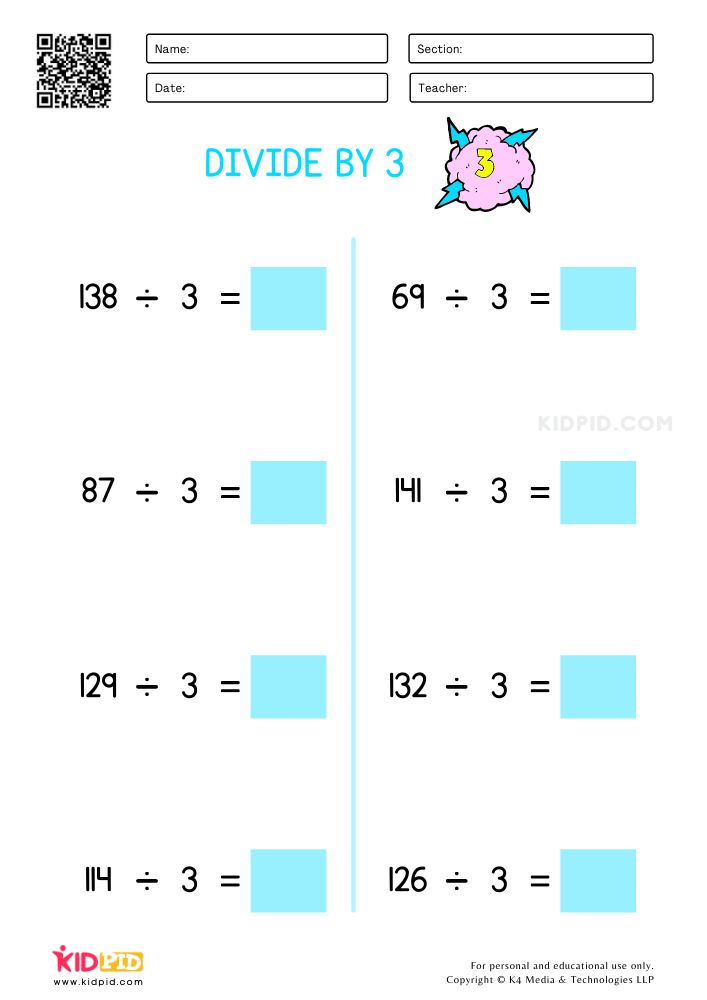The more one practices the more he retains. Hence, we provide six different sheets in the worksheet each of which contains eight different questions. In total there are forty-eight questions for students to practice the process of division. Make sure to provide other exercises for the kid to fully understand the process. You should also keep in mind that there is no need to rush students while trying to teach. Do not burden them with all these at once if the student is having a problem solving these.

## Introduction to Division

Contents What Is A Division? A division basically is to divide any number into the required given equal parts. A division is generally the opposite…

## Colorful Rainbow Crafts for Kids

Craftwork is not limited to kids, in fact, it’s not confined to any age limit. By doing craftwork our creativity increases. We are in school,…

## Animal Division Printable Worksheets

Now that your kid has had enough practice with addition and subtraction, it’s time to take things to the next level. This week we bring…

## Easy Beach Crafts For Kids

A fun day trip for the whole family is going to the beach! Everyone enjoys playing on the beach, swimming in the water, and basking…

## Single-digit Addition Math Worksheets & Free Printables

We have bought single-digit addition math foundational worksheets that will help your kid in building and brushing their counting skills. In this busy world, it…

+